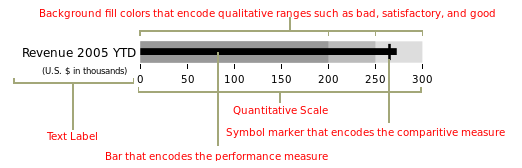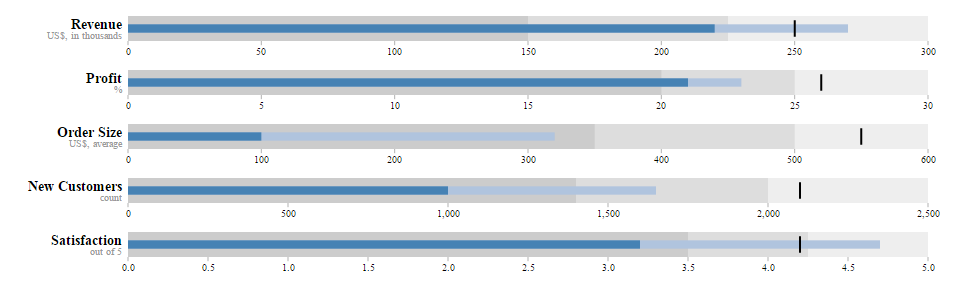/ Charts

# Bullet Graphs using R

I came across this library for creating Bullet graphs using R.

Installation of the package is straightforward:

``````library(devtools)
install_github(c('rstudio/htmltools',
'ramnathv/htmlwidgets',
'sipemu/d3Dashboard'))
``````

The example bullet graph can be created as follows:

``````require("d3Dashboard")

ytd2005 <- list(
title=list("Revenue", "Profit", "Order Size", "New Customers", "Satisfaction"),
subtitle=list("US\$, in thousands", "%", "US\$, average", "count", "out of 5"),
range=list(c(150, 225, 300),
c(20, 25, 30),
c(350, 500, 600),
c(1400, 2000, 2500),
c(3.5, 4.25, 5)),
measures=list(c(220, 270),
c(21, 23),
c(100, 320),
c(1000, 1650),
c(3.2, 4.7)),
markers=list(250, 26, 550, 2100, 4.2)
)

# Plot
bulletGraph(ytd2005)
``````

After reading the wiki, it is interesting about the information that can be gleaned from the bullet graph.• The dark center line represents the actual value.
• The dark vertical line represents a target value.
• The colored bands represent ranges, such as poor, average, and good.